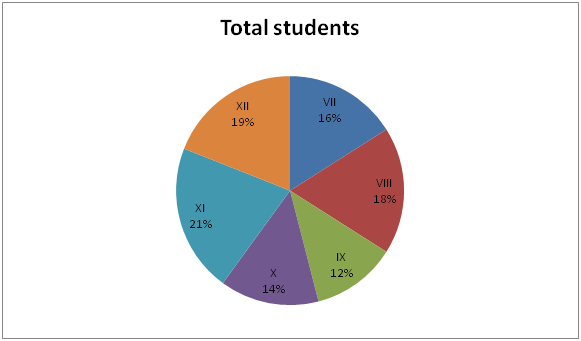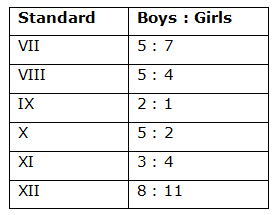# CWC/FCI Prelims 2019 – Quantitative Aptitude Questions (Day-20)

Dear Readers, Exam Race for the Year 2019 has already started, To enrich your preparation here we are providing new series of Practice Questions on Quantitative Aptitude – Section for CWC/FCI Exam. Aspirants, practice these questions on a regular basis to improve your score in aptitude section. Start your effective preparation from the right beginning to get success in upcoming CWC/FCI Exam.

[WpProQuiz 5619]

1) If 4 men or 8 women or 6 boys can finish a work in 60 days working 9 hours a day. The number of days taken by 16 men, 6 women and 12 boys working 6 hours a day will be?

a) 12 days

b) 14 (2/5) days

c) 11 days

d) 13 (1/3) days

e) None of these

2) A boat can cover 18 km downstream in 45 minutes. If the speed of the current is one-third of the speed of boat in still water, then how much distance can the boat cover upstream in 54 minutes?

a) 13.2 km

b) 16 km

c) 18 km

d) 10.8 km

e) None of these

3) A, B and C started a business with their investments in the ratio 3: 5: 4. After 6 month, A invested the half amount more as before and B invested same the amount as before while C withdrew (1/4)th of his investment. Find the ratio of their profits at the end of the year

a) 9: 11: 7

b) 15: 30: 14

c) 12: 15: 8

d) 33: 37: 29

e) None of these

4) Mani spends 32% of the monthly income on Food and 15 % of the income on education of his children and 35 % of the remaining income on entertainment, now he is left with Rs. 24115. Find the monthly income of Mani

a) Rs. 70000

b) Rs. 65000

c) Rs. 60000

d) Rs. 55000

e) None of these

5) Average of the five consecutive numbers is 29. Find the sum of the largest and smallest number?

a) 62

b) 66

c) 58

d) 60

e) None of these

Directions (Q. 6 – 10): Study the following information carefully and answer the given questions:

The following pie chart shows the total number of students studied in different standards and the table shows the ratio of boys to that of girls among them.

Total number of students = 30006) Find the difference between the total number of boys studied in standard VII to that of total number of girls studied in standard XI?

a) 210

b) 150

c) 160

d) 190

e) None of these

7) Find the ratio between the total number of students studied in standard X to that of total number of girls studied in standard XII?

a) 14: 11

b) 22: 17

c) 19: 15

d) 23: 16

e) None of these

8) Find the central angle for standard IX?

a) 56.50

b) 48.70

c) 52.40

d) 43.20

e) None of these

9) Find the average number of boys studied in standard VIII, X and XII together?

a) 265

b) 280

c) 295

d) 320

e) None of these

10) Total number of students studied in standard VII is what percentage of total number of girls studied in standard VIII?

a) 190 %

b) 240 %

c) 200 %

d) 165 %

e) None of these

4 men = 8 women

8 women = 6 boys

16 men and 6 women and 12 boys

= > 32 women + 6 women + 16 women = 54 women

Women      days           hours

8                 60               9

54                ?                  6

(8*60*9) = (54* x days *6)

X = 40/3 = 13 (1/3) days

The speed of downstream= D/T = 18/(45/60) = 24 km/hr

The speed of the current = (1/3)*the speed of boat in still water

Speed of boat: Speed of Current = 3: 1 (3x, x)

Speed of downstream = Speed of boat + Speed of Current = 4x

24 = 4x

X= 6

Speed of boat = 3x = 18 km/hr, Speed of Current = x = 6 km/hr

Speed of upstream = Speed of boat – Speed of Current = 2x

Speed of upstream = 12 km/hr

Time = 54 min = (54/60) hr

Distance = S*T = 12*(54/60) = 10.8 km

The share of A, B and C

= > [3x*6 + 3x*(3/2)*6]: [5x*6 + 10x*6]: [4x*6 + (4x*(3/4)*6]

= > [18x + 27x]: [30x + 60x]: [24x + 18x]

= > 45x: 90x: 42x

= > 15: 30: 14

Let the monthly income be x,

According to the question,

X*(53/100)*(65/100) = 24115

X = 24115*(100/53)*(100/65) = Rs. 70000

Average of the five consecutive numbers = 29

Sum of five consecutive numbers = 29*5 = 145

X + x + 1 + x + 2 + x + 3 + x + 4 = 145

5x + 10 = 145

5x = 135

X = 27

Required sum = (27 + 4) + 27 = 58

Direction (6-10) :

The total number of boys studied in standard VII

= > 3000*(16/100)*(5/12) = 200

The total number of girls studied in standard XI

= > 3000*(21/100)*(4/7) = 360

Required difference = 360 – 200 = 160

The total number of students studied in standard X

= > 3000*(14/100) = 420

The total number of girls studied in standard XII

= > 3000*(19/100)*(11/19) = 330

Required ratio = 420: 330 = 14: 11

The central angle for standard IX

= > (12/100)*360 = 43.20

The total number of boys studied in standard VIII, X and XII together

= > 3000*(18/100)*(5/9) + 3000*(14/100)*(5/7) + 3000*(19/100)*(8/19)

= > 300 + 300 + 240 = 840

Required average = 840/3 = 280

Total number of students studied in standard VII

= > 3000*(16/100) = 480

Total number of girls studied in standard VIII

= > 3000*(18/100)*(4/9) = 240

Required % = (480/240)*100 = 200 %